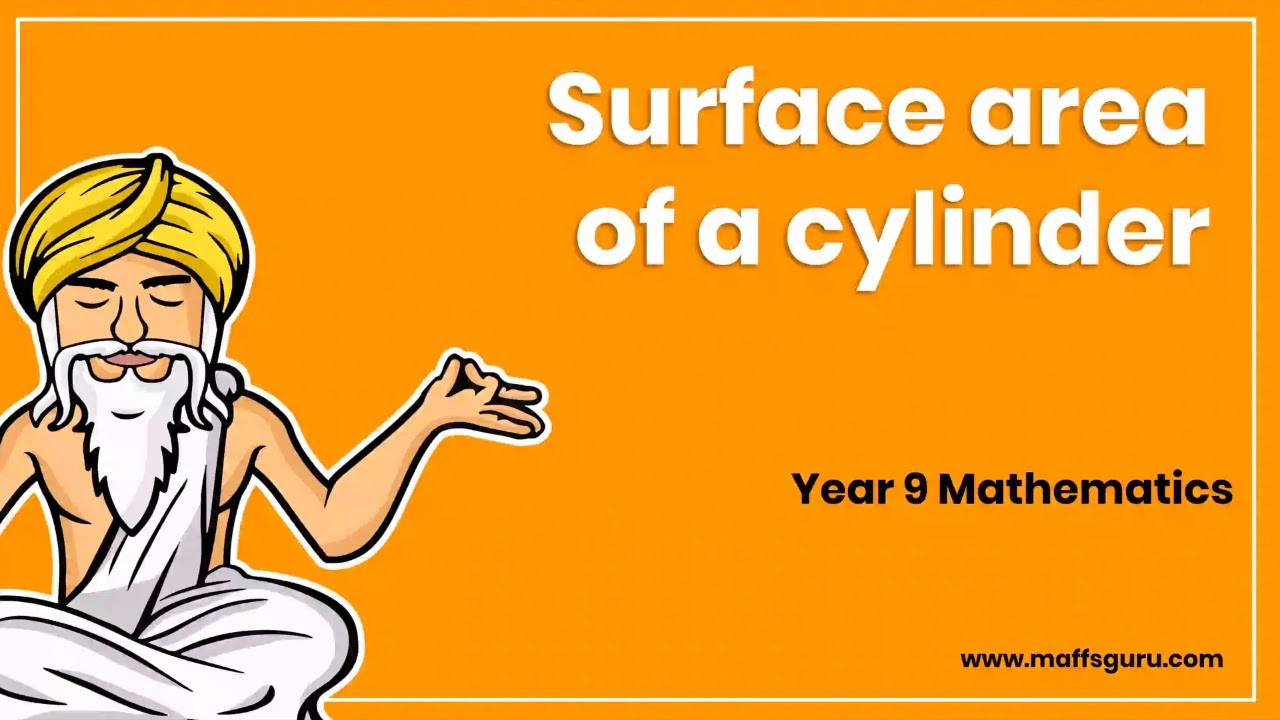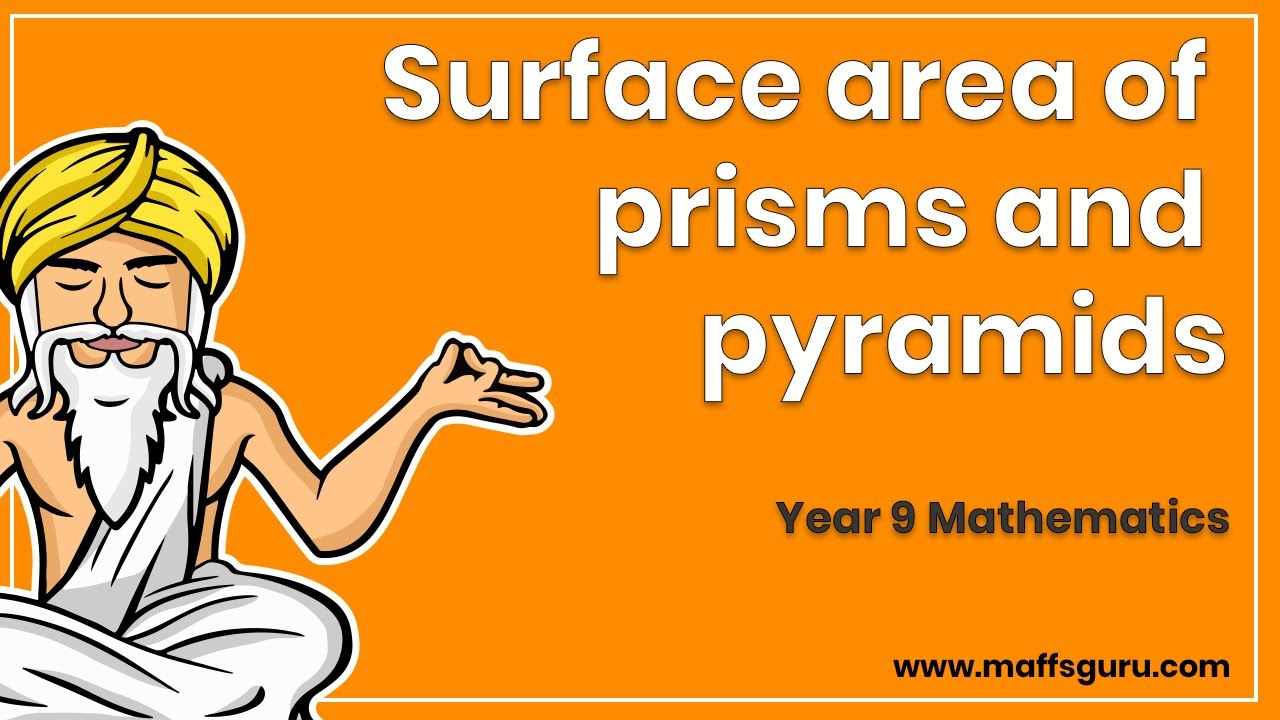### Latest Videos

These are the latest videos which have been posted to MaffsGuru.com.
There are always new videos being added every day.
Videos which are greyed out are for subscribers only.
Please consider helping support me create these videos by taking out a very cheap subscription. Click here

01

## Essential Mathematics (Year 10) Surface area and volume of spheres (10A)Essential Mathematics (Year 10)

02

03

## Further Mathematics: Units 3 and 4 Non-linear graphsFurther Mathematics: Units 3 and 4

04

## Further Mathematics: Units 3 and 4 Step graphsFurther Mathematics: Units 3 and 4

05

## Further Mathematics: Units 3 and 4 Line segment graphsFurther Mathematics: Units 3 and 4

06

## Further Mathematics: Units 3 and 4 The general equation of a straight lineFurther Mathematics: Units 3 and 4

07

## Essential Mathematics (Year 9) Surface area of a cylinderEssential Mathematics (Year 9)

08

## Essential Mathematics (Year 9) Surface area of prisms and pyramidsEssential Mathematics (Year 9)